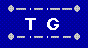The Quadratic Formula in Verse Writings index       Home

The Quadratic Formula is the general solution to an equation of the form
Ax2 + Bx + C = 0

 First you subtract from the square of B, ... the product of four times A times C. The next thing which you must compute, ... is from that, the exact square root. Add or subtract it to minus B, ... we're almost done as you shall see. Divide it all by two times A, ... and the answers are yours, hip hip hooray! The formula is -B ± √(B2 - 4 A C) -----------------------             2 A